• 时间复杂度和空间复杂度 这是任何AI工程师必须要深入理解的概念。对于每一个设计出来的算法都需要从这两个方面来分析 O(N), O(N^2) : o notation #%% int a = 0, b = 0; for (i = 0; i < N; i++) { # O(N)+O(N)=...
• 一、引言:写给自己看算法是程序的灵魂，想学好算法就必须先搞懂时间复杂度时间复杂度就是算基本语句条数5个例题二、例题1)、for(int i=0; ifor(int j=i; jSystem.out.println("执行一次");}}i=0时，j=0，j循环执行...
一、引言:写给自己看算法是程序的灵魂，想学好算法就必须先搞懂时间复杂度算时间复杂度就是算基本语句条数5个例题二、例题1)、for(int i=0; ifor(int j=i; jSystem.out.println("执行一次");}}i=0时，j=0，j循环执行n次i=1时，j=1，j循环执行n-1次i=2时，j=2，j循环执行n-2次推广到i=n-1时，j=n-1，j循环执行1次Sn=n+n-1+n-2+...+1=n×(n+1)/2时间复杂度为：O(n2)2)、i=0;while((i+1)*(i+1)<=n){i++;}第1次，i=1第2次，i=2推广到第x次，i=x设第x次退出循环，则(i+1)×(i+1)>nx=i>(√n)-1 次时间复杂度为：O(√n)3)、int i=1;while(ii = i*2;}第1次：i=21第2次：i=22第3次：i=23......第x次：i=2x设第x次退出循环，则有i=2x ≥ n取对数解得x=logn，共执行logn次时间复杂度为：O(logn)4)、for(int i=1; i<=n; i++){for(int j=1; j<=i; j++){for(int k=1; k<=j; k++){System.out.println("执行一次");}}}粗略的估计:最外层i循环n次，j循环最坏的情况执行n次，k循环最坏的情况也执行n次，即时间复杂度为O(n3)详细计算:i=1时，j、k循环都只执行一次，输出语句执行1次i=2时，j循环[1,2]执行2次，每一次分别有一个k循环，分别是[1,1]、[1,2]输出语句执行1+2次i=3时，j循环[1,3]执行3次，每一次分别有一个k循环，分别是[1,1]、[1,2]、[1,3]输出语句执行1+2+3次可以推广到i=n时，j循环[1,n]执行n次，每一次分别有一个k循环，分别是[1,1]、[1,2]......[1,n]输出语句执行1+2+......+n次总共:Sn=(1)+(1+2)+(1+2+3)+......+(1+2+3+...+n)1~n项和取n2放大一点看就是n个这样的n2相加，即n3时间复杂度为：O(n3)5)、for(int i=0; ifor(int j=2*i; jSystem.out.println("执行一次");}}粗略的估计i=0时，j=0，内循环n次即时间复杂度为O(n2)详细计算i=0时，j=0，[0,n)内循环n次i=1时，j=2，[2,n)内循环n-2次i=2时，j=4，[4,n)内循环n-4次推广到i=(n/2)-1，j=n-2，[n-2,n)内循环2次i=n/2时，j=n，跳出循环综上Sn=n+n-2+n-4+...+2共n/2项，首项为n，公差-2Sn=(((n/2)×[(n/2)-1)]×(-2)/2) + (n/2)×n输出语句执行了(n2+2n)/4次时间复杂度为：O(n2)三、总结一定要学会算时间复杂度很重要很重要很重要
展开全文• #%% md 1. 简单的复杂度的回顾 #%% def compute(a,b): n,s = 10,0 for i in range(n): for j in range(n): s = s + a * b return s 时间复杂度：O(N^2)， 空间复杂度：O(1...2. 归并排序以及时间复杂度以及Mas...
#%% md
1. 简单的复杂度的回顾
#%%

def compute(a,b):
n,s = 10,0
for i in range(n):
for j in range(n):
s = s + a * b
return s
时间复杂度：O(N^2)， 空间复杂度：O(1)

#%% md

2. 归并排序以及时间复杂度以及Master Thereom
#%%
#%% md
3. Fibonanci number (斐波那契数)
序列一次为 1，1，2，3，5，8，13，21，…
问题： 怎么求出序列中第N个数？
#%%

T(n) = T(n-2) + T(n-1)

def fib(n):
# base case
if n < 3:
return 1
return fib(n-2)+fib(n-1)
print (fib(50))

#%%

int fib( int num ){  //递归版本
if(num <= 2){  //边界问题很重要
return 1;
}
return fib(num-1) + fib(num-2);
}

int fib_arr(int num){ // 这个数是下标+1
int arr[num];
int i = 2;
arr = 1;
arr = 1;
while(i <= num-1){
arr[i] = arr[i-1] + arr[i-2];
i++;
}

return arr[num-1];
}

int main(){
int c = fib(6);
cout << c;
cout << "\n";
c = fib_arr(6);
cout << c;
return 0;
}

时间复杂度？
import numpy as np
def fib(n):
tmp = np.zeros(n)
tmp = 1
tmp = 1
for i in range(2,n):
tmp[i] = tmp[i-2]+tmp[i-1]

return tmp[n-1]

O(N) O(2^n)

def fib(n):
tmp = np.zeros(n)
tmp = 1
tmp = 1
for i in range(2,n):
tmp[i] = tmp[i-2]+tmp[i-1]

return tmp[n-1]

def fib(n):
a,b=1,1
c =0
for i in range(2,n):
c = a + b
a = b
b = c
return c

思考题： 怎么在O(1)的时间复杂度下计算FIB(N)？
套公式（组合数学递推公式）
思考题： 这个公式怎么得来的？
提示： 转换成矩阵的连乘的形式， 矩阵连乘可以简化（MATRIX DECOMPOSION）
#%% md
#%%


展开全文算法
• 文中 红色标记的为重点非常有用的东西，一定要...这里的 reverse 函数中交换了多少次与s有多少位有关，即位数除以2，s的位数由于循环的次数有关，循环次数就是 O(logn），所以为log n / 2， 所以时间复杂都是log n...
文中 红色标记的为重点非常有用的东西，一定要牢记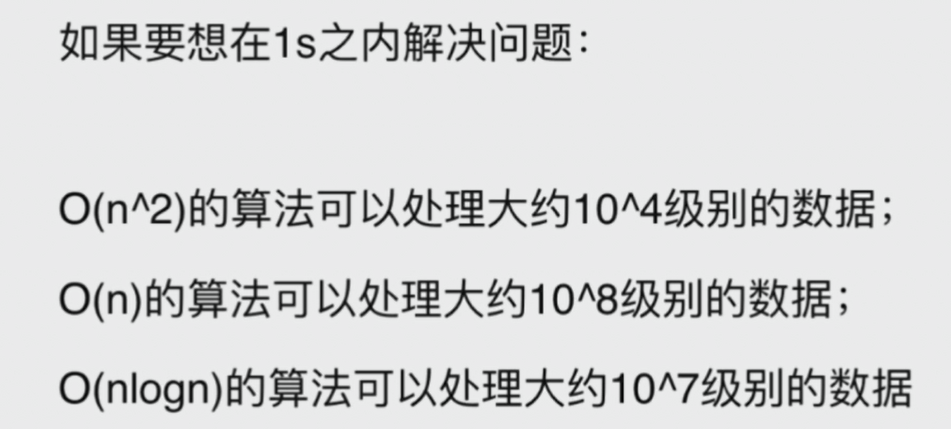空间复杂度：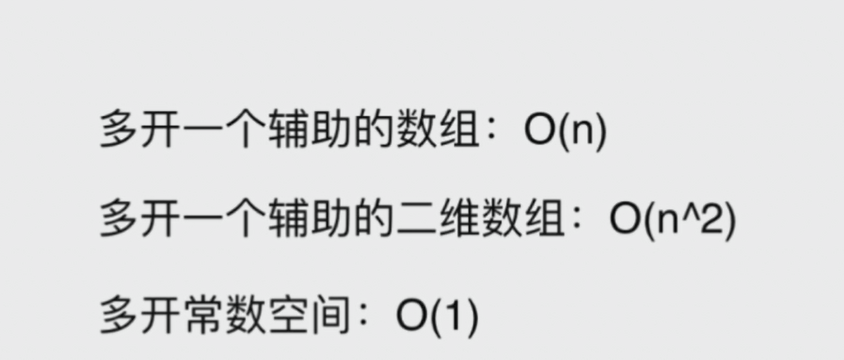递归的调用时有空间代价的：例如下面的是:递归调用深度是n级别的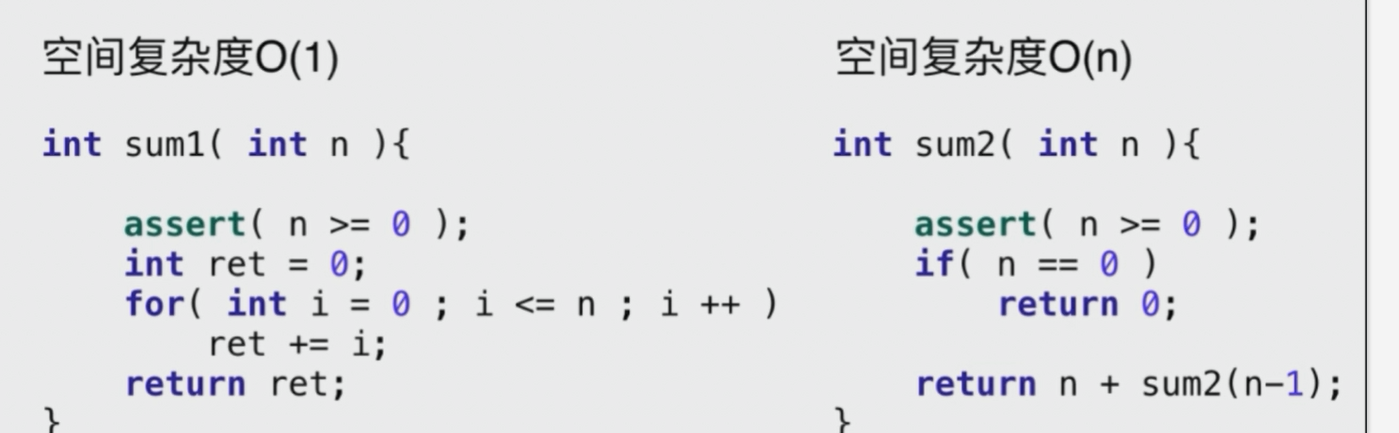常见复杂度分析：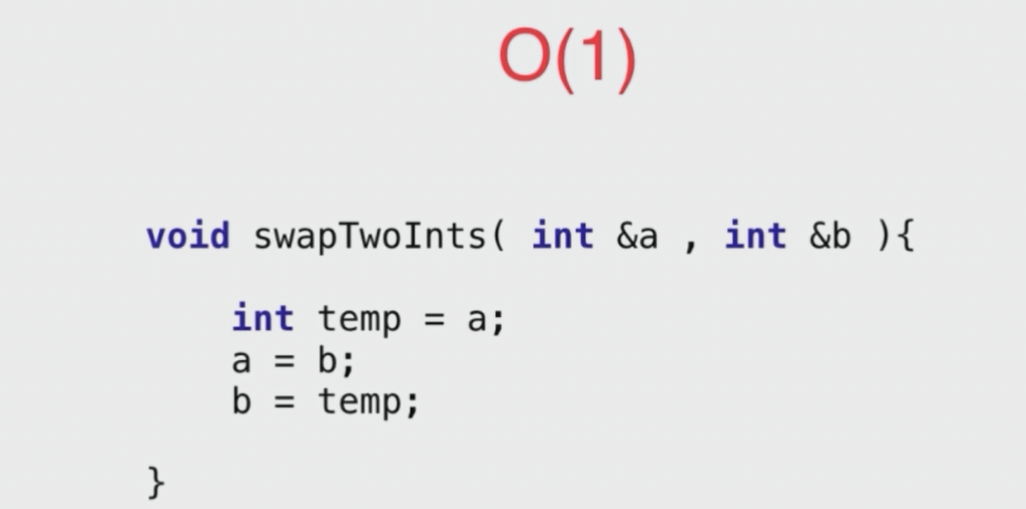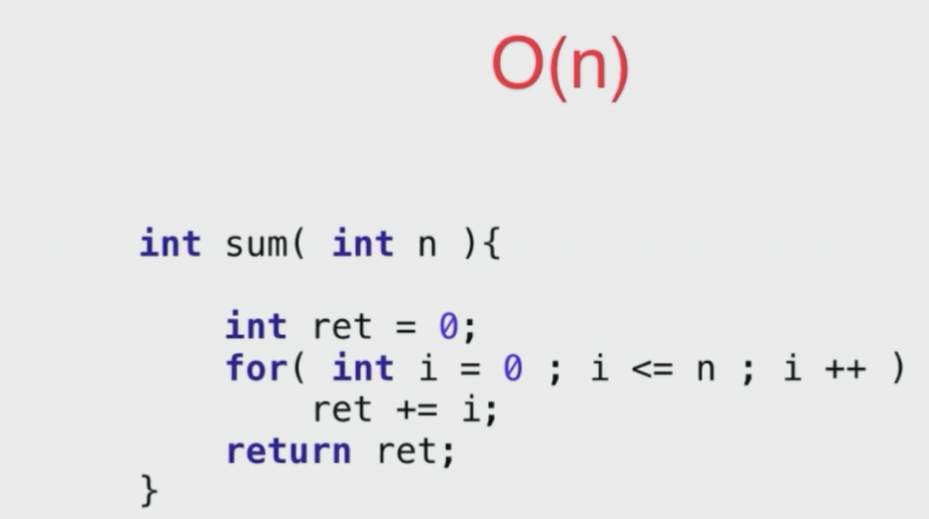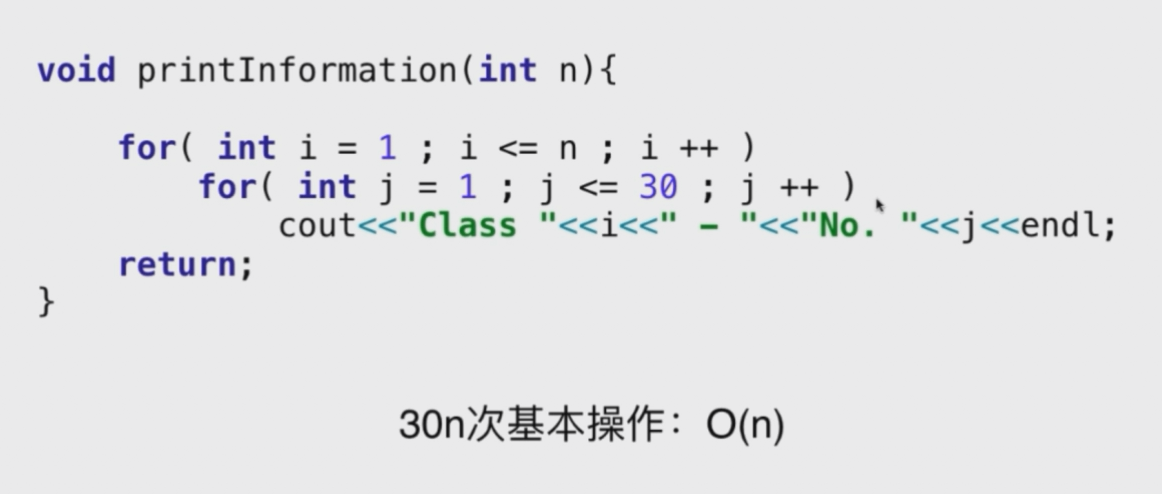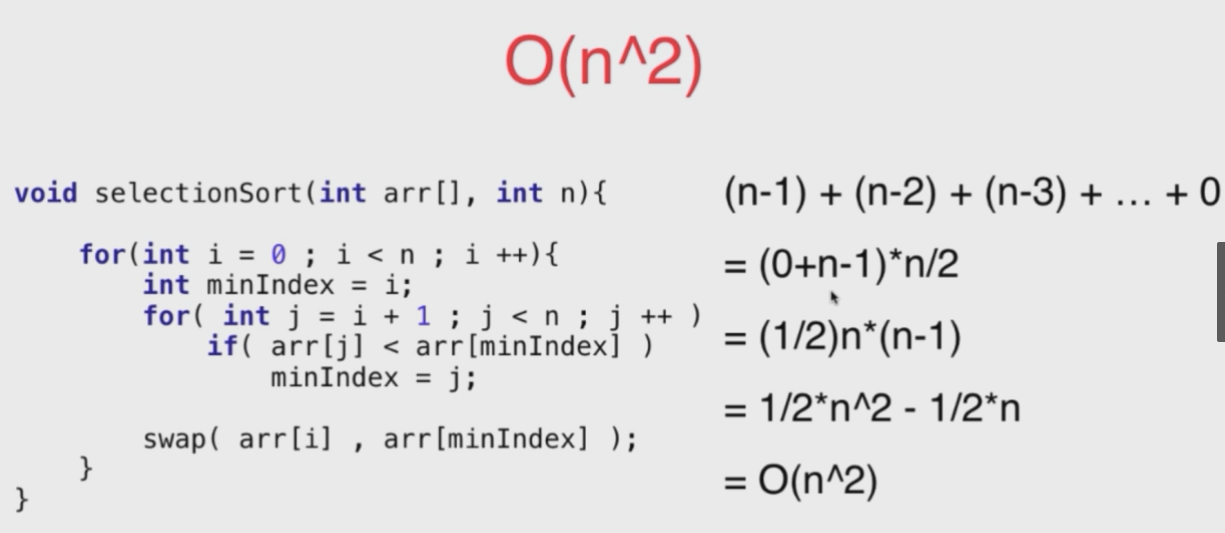选择排序

二分查找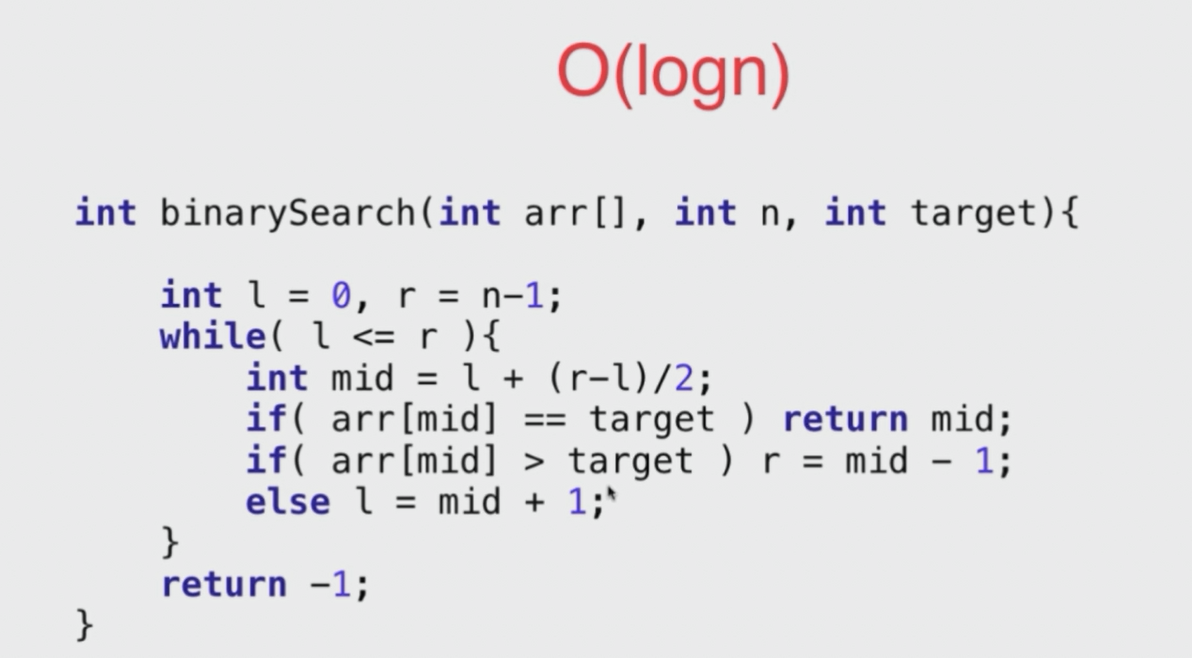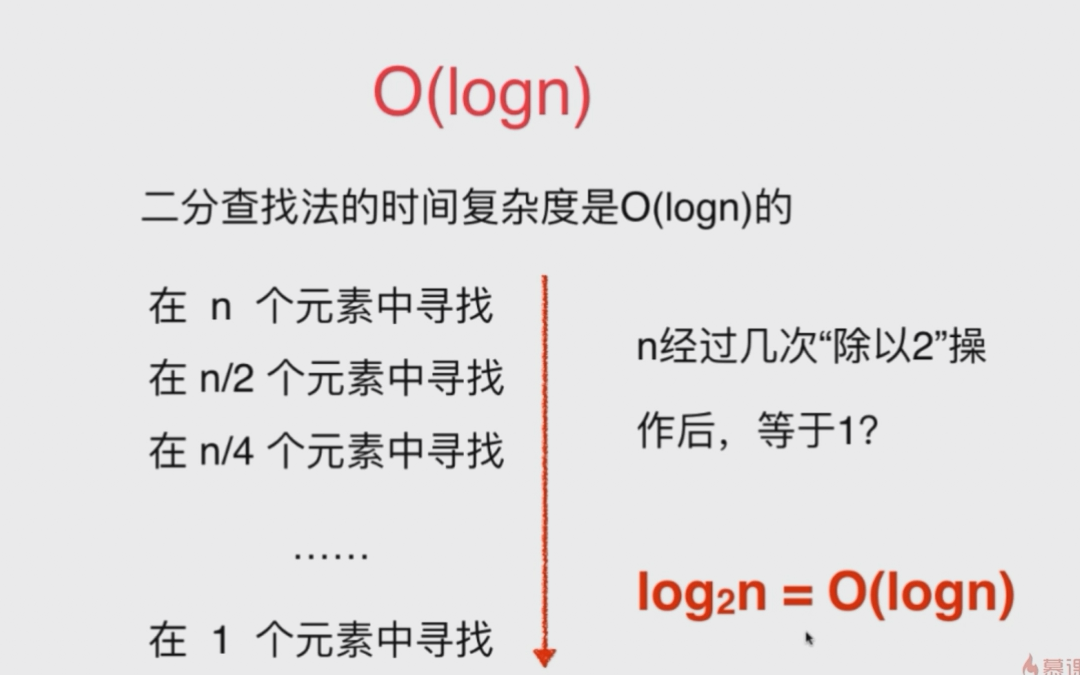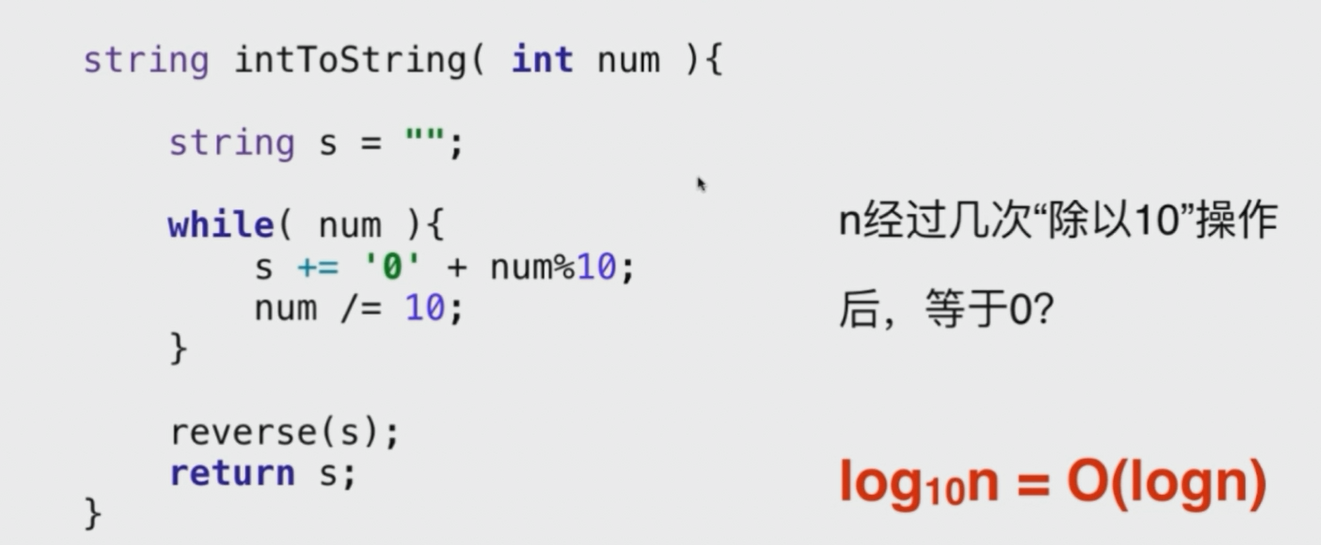这里的 reverse 函数中交换了多少次与s有多少位有关，即位数除以2，s的位数由于循环的次数有关，循环次数就是 O(logn），所以为log n / 2，  所以时间复杂都是log n .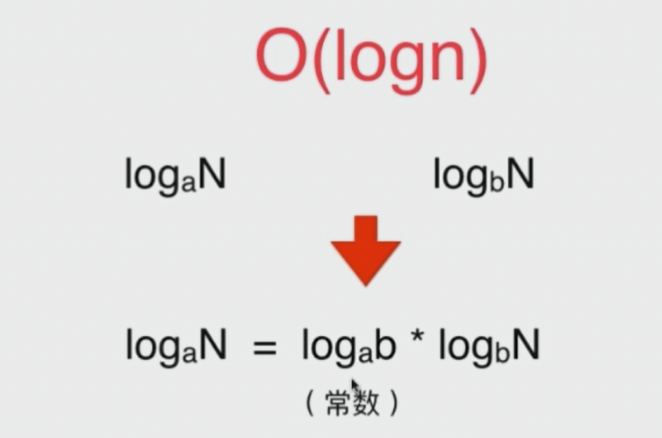数学中这个公式告诉我们，对数 的底 对算法复杂度 不重要，因为只差一个常数（重点）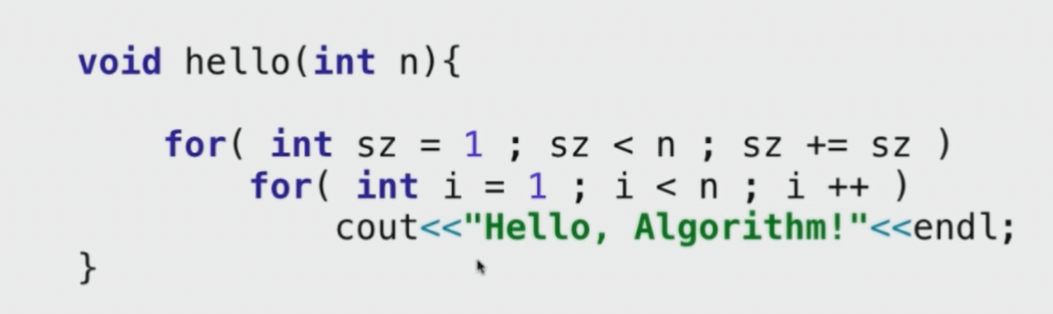复杂度是nlogn（重点）外层循环可以理解为 1 不断乘以2，至到等于n 所以时logn,内层循环是 n 所以是nlogn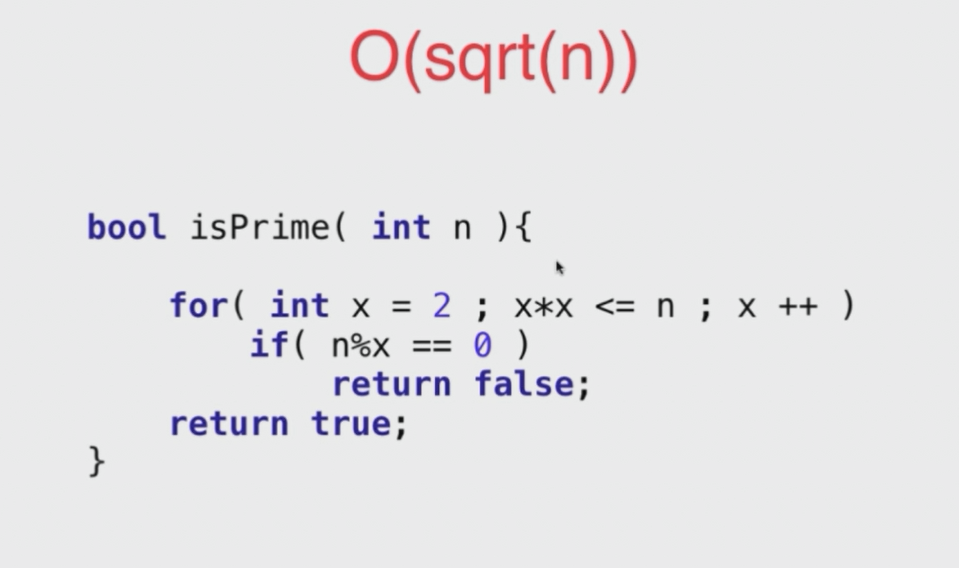递归算法的复杂度分析：

首先并不是所有的递归算法的复杂度都是nlogn，需要根据具体的情况进行分析（重点）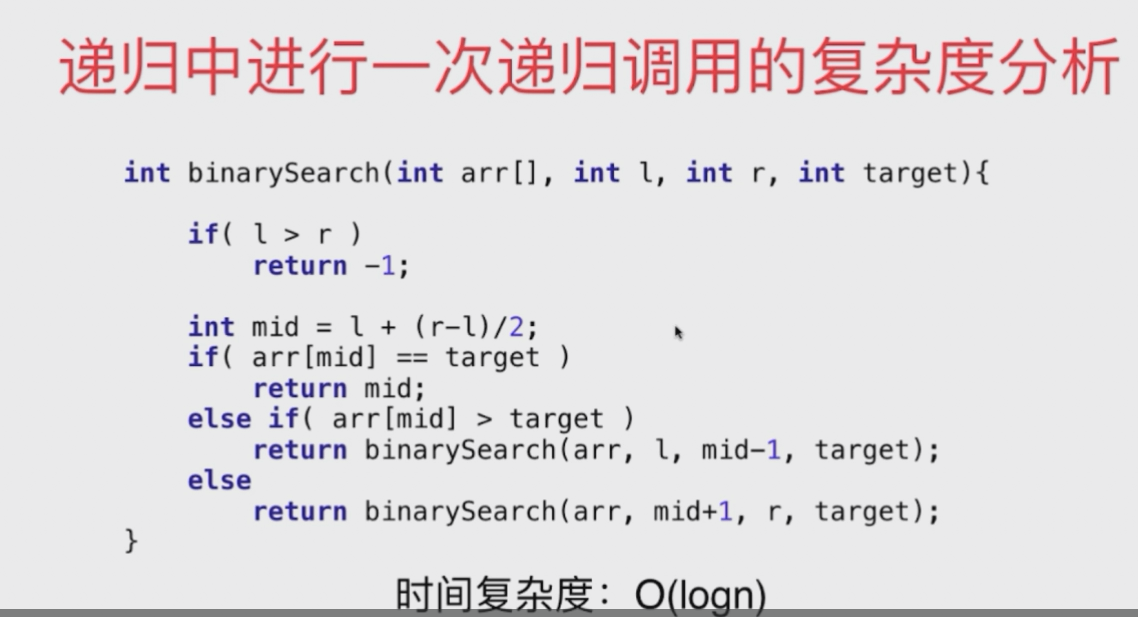即我们可以总结为

如果递归函数中，只进行一次递归调用，递归深度为depth，在每个递归函数中，时间复杂度为T，则总体的时间复杂度为O(T * depth)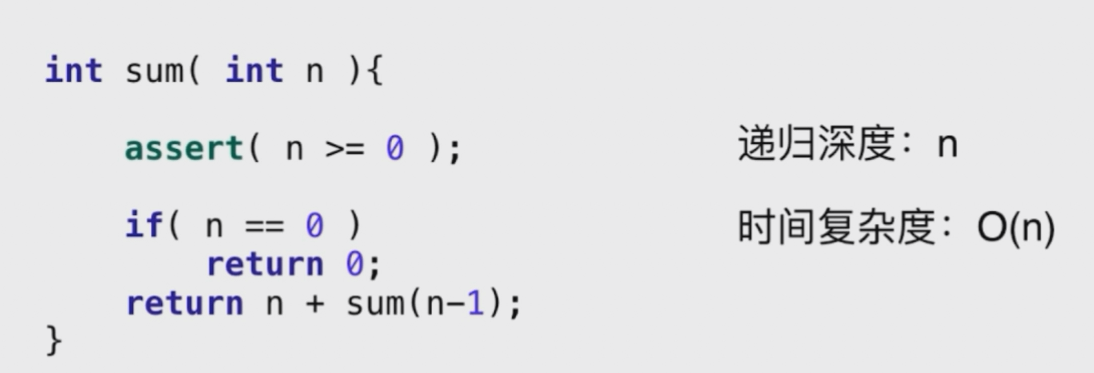幂运算的算法：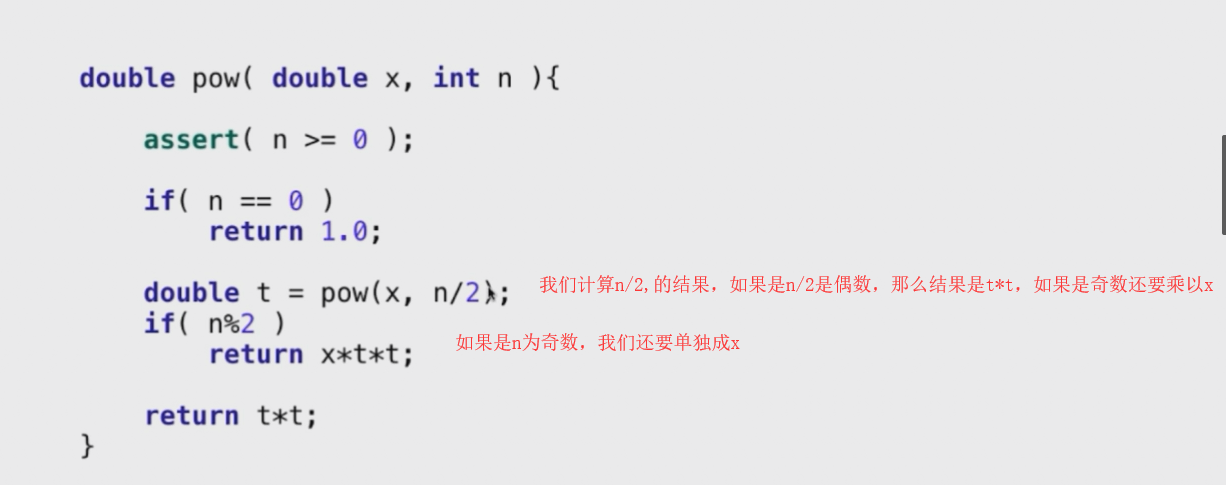时间复杂度是log n

如果递归算法中出现多次递归调用，下面以两次递归调用为例子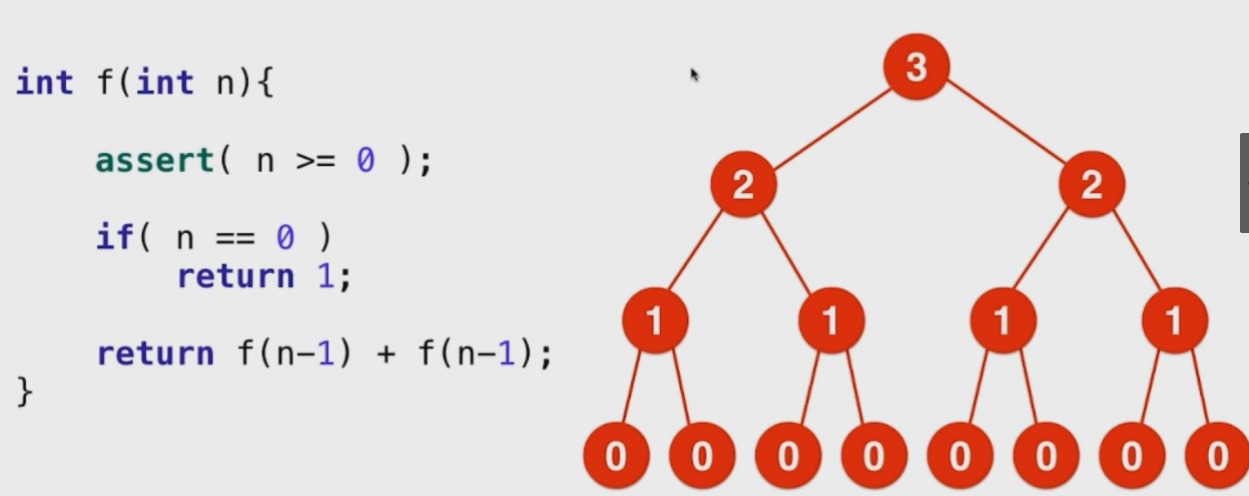以上就是两次递归为列，我们画出递归调用树，查出所有的节点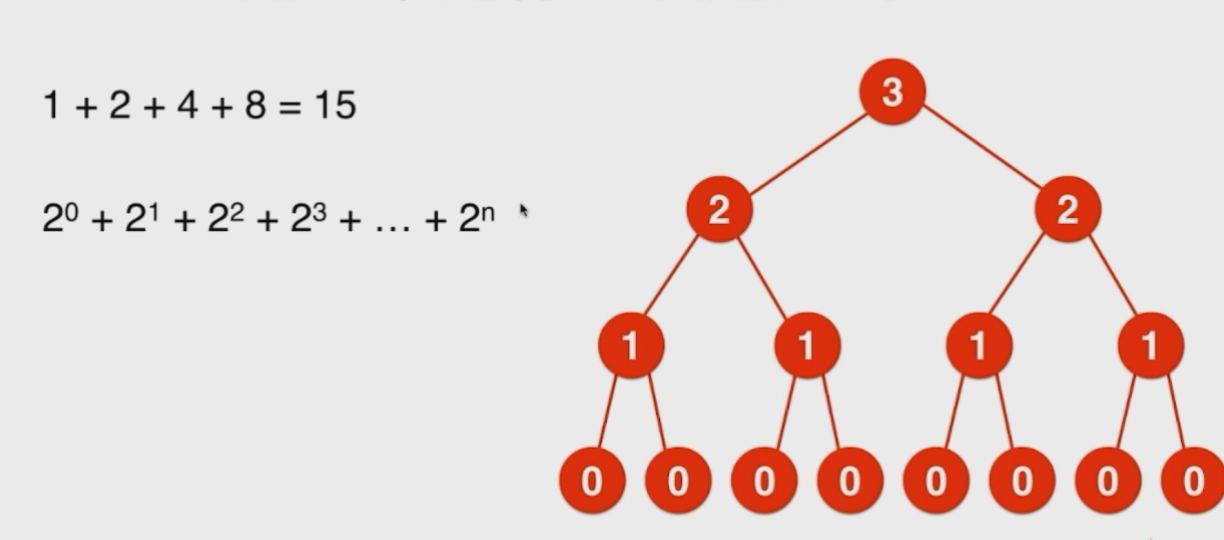即是O(2^n)方 的算法，这个是指数级的算法，

归并排序算法（底层用的也是递归）但是算法复杂度是 O(nlogn)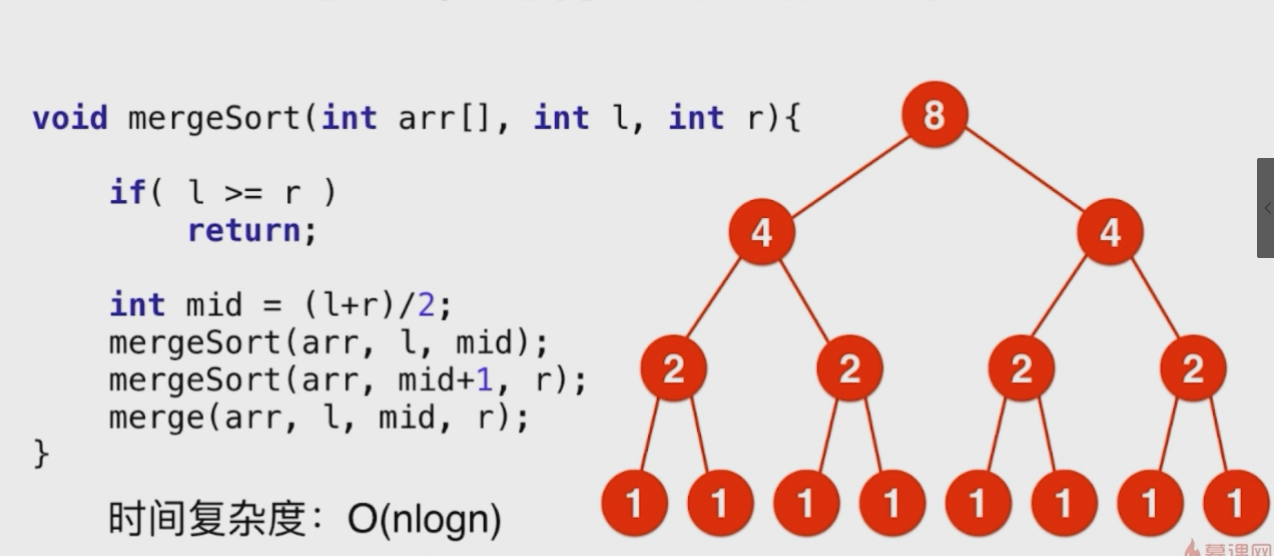我们可以理解为每层排序的数 的个数一直没有变，而深度是log n

所以是n logn

递归调用的算法时间复杂度的 各种情况：

主定理。注定里是一个式子，归纳了递归时间复杂度的所有情况，一个函数把数据分为几分进行递归，每一份的时间复杂度 是多少，都可以查看一下。


展开全文• 时间复杂度和空间复杂度前言算法效率时间复杂度1.时间复杂度的概念2.大O的渐进（估算）表示法推导大O阶方法(重点！！！)：另外有些算法的时间复杂度存在最好、平均、和最坏情况（重点！！！）：3.常见时间复杂度计算...
时间复杂度和空间复杂度前言算法效率时间复杂度1.时间复杂度的概念2.大O的渐进（估算）表示法推导大O阶方法(重点！！！)：另外有些算法的时间复杂度存在最好、平均、和最坏情况（重点！！！）：3.常见时间复杂度计算举例实例1：实例2：实例3实例4：实例5：空间复杂度1.空间复杂度的概念2.常见空间复杂度计算举例实例1：实例2：
前言
时间复杂度和空间复杂度的求解可以说是所有数据结构初学者所面对的第一个重点，它是衡量一段代码优劣的重要指标。本篇文章博主会详细的向大家介绍求解一段代码时间复杂度和空间复杂度的方法，并且会举例来说明，希望大家能够静下心慢慢的看完，相信一定会对大家带来帮助。

算法效率
刚刚我提到过，时间复杂度和空间复杂度是衡量一段代码优劣的重要指标，其实主要影响的就是算法的效率，所以接下来我想先请大家了解一下算法效率的概念。

算法效率分析分为两种：第一种是时间效率，第二种是空间效率。时间效率被称为时间复杂度，而空间效率被称作空间复杂度。时间复杂度主要衡量的是一个算法的运行速度，而空间复杂度主要衡量一个算法所需要的额外空间，在计算机发展的早期，计算机的存储容量很小。所以对空间复杂度很是在乎。但是经过计算机行业的迅速发展，计算机的存储容量已经达到了很高的程度。所以我们如今已经不需要再特别关注一个算法的空间复杂度。

了解完算法效率的概念之后，接下来我们就来看看时间复杂度是如何求解的。
时间复杂度
1.时间复杂度的概念

在计算机科学中，算法的时间复杂度是一个函数（这里指的是数学上的函数），他定量描述了该算法的运行时间。一个算法执行所耗费的时间，从理论上说，是不能算出来的，只有你把程序放在机器上跑起来，才能知道。但是我们需要每个算法都上机测试吗？是可以都上机测试，但是这很麻烦，所以才有了时间复杂度这个分析方式。一个算法所花费的时间与其中语句的执行次数成正比例，算法中基本操作的执行次数，为算法的时间复杂度。

2.大O的渐进（估算）表示法
问：请计算一下Func1基本操作执行了多少次？
void Func1(int N)
{
int count = 0;
for (int i = 0; i < N; ++i)
{
for (int j = 0; j < N; ++j)
{
++count;
}
}
for (int k = 0; k < 2 * N; ++k)
{
++count;
}
int M = 10;
while (M--)
{
++count;
}
printf("%d\n", count);
}

Func1执行的基本操作次数：F(N) = N² + 2*N + 10
N = 10 F(N) = 130
N = 100 F(N) = 10210
N = 1000 F(N) = 1002010
实际上我们计算时间复杂度的时候，其实并不一定要计算精确的执行次数，而只需要大概执行次数，那么这里我们使用大O的渐进表示法。

大O符号（Big O notation）:是用于描述函数渐进行为的数学符号。

推导大O阶方法(重点！！！)：

1.用常数1取代运行时间中的所有加法常数。
2.在修改后的运行次数函数中，只保留最高阶项。
3.如果最高阶项存在且不是1，则去除与这个项目相乘的常数。得到的结果就是大O阶。

使用大O渐进表示法以后，根据规则第二点只保留最高项，Func1的时间复杂度为：O（N²）
N = 10 F(N) = 100
N = 100 F(N) = 10000
N = 1000 F(N) = 1000000
通过上面我们会发现大O的渐进表示法去掉了那些对结果影响不大的项，简洁明了的表示出了执行次数。
另外有些算法的时间复杂度存在最好、平均、和最坏情况（重点！！！）：
最坏情况：任意输入规模的最大运行次数（上界）
平均情况：任意输入规模的期望运行次数
最好情况：任意输入规模的最小运行次数（下界）
例如：在一个长度为N的数组中搜索一个数据x
最好的情况：1次找到
最坏的情况：N次找到
平均情况：N/2次找到

在实际情况中时间复杂度的估算实际上是一种悲观的估算，关注的是算法的最坏运行情况，所以数组中搜索数据的时间复杂度复杂度为O（N）。

3.常见时间复杂度计算举例
实例1：
// 计算Func2的时间复杂度？
void Func2(int N)
{
int count = 0;
for (int k = 0; k < 2 * N; ++k)
{
++count;
}
int M = 10;
while (M--)
{
++count;
}
printf("%d\n", count);
}

本段代码基本执行操作是2N+10次，根据推导大O阶方法，只保留最高阶并且去除与这个项目相乘的常数，时间复杂度为O（N）
实例2：
// 计算Func3的时间复杂度？
void Func3(int N, int M)
{
int count = 0;
for (int k = 0; k < M; ++k)
{
++count;
}
for (int k = 0; k < N; ++k)
{
++count;
}
printf("%d\n", count);
}

本段代码基本执行操作是M + N次，因为M和N是两个未知数，所以时间复杂度为O（M + N）.
这里多提一点，如果告诉M远大于N，那么此时的时间复杂度就为O(M)，因为时间复杂度就是一个估算的过程。
实例3
// 计算Func4的时间复杂度？
void Func4(int N)
{
int count = 0;
for (int k = 0; k < 100; ++k)
{
++count;
}
printf("%d\n", count);
}

本段代码基本执行次数为100次，100是一个常数，根据推导大O阶方法，用常数1取代常数，时间复杂度为O(1)。
实例4：
// 计算strchr的时间复杂度？
const char * strchr(const char * str, int character);

这里简单介绍一下strchr函数，这个函数是在一个字符串中查找一个字符。
这样的话基本执行操作次数最好的情况是1次直接找到，最坏的情况是N次遍历完整个字符串才找到，而时间复杂度关注的是最坏的情况，故时间复杂度为O(N)。
实例5：
// 计算斐波那契递归Fibonacci的时间复杂度？
long long Fibonacci(size_t N)
{
return N < 2 ? N : Fibonacci(N - 1) + Fibonacci(N - 2);
}

这道题如果仅仅看函数的话是分析不出来的，这里需要画图来分析。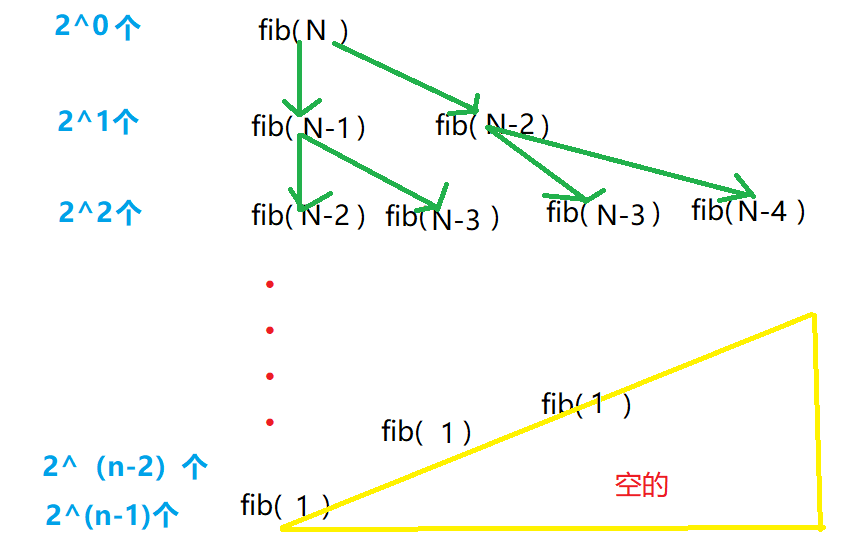总共的调用次数应该是1+21+22+···+2(n-2)+2(n-1)-空掉部分 = 2n - 1 - 空掉部分。因为有的地方会提前算到1而结束，所以必须减掉一部分。
根据推导大O阶方法，最终的时间复杂度应该是O（2^n）。
介绍这道题的主要目的是想告诉大家，在求解时间复杂度的时候千万不要仅仅抱着代码在那啃。要知道很多复杂程序的时间复杂度光用眼睛看代码是看不出来的，毕竟人脑是有限的，所以需要自己动手画图，这样才能看清楚程序到底运行了多少次数，因此大家一定要养成勤动手画图的好习惯。
时间复杂度的求解就介绍到这里，下面我们再一起来看空间复杂度的求解。
空间复杂度
1.空间复杂度的概念

空间复杂度是对一个算法在运行过程中临时占用存储空间大小的量度。空间复杂度不是程序占用了多少字节的空间，因为这个也没太大意义，所以空间复杂度算的是变量的个数。空间复杂度计算的规则基本跟时间复杂度类似，也使用大O渐进表示法。

2.常见空间复杂度计算举例
实例1：
// 计算BubbleSort的空间复杂度？
void BubbleSort(int* a, int n)
{
assert(a);
for (size_t end = n; end > 0; --end)
{
int exchange = 0;
for (size_t i = 1; i < end; ++i)
{
if (a[i - 1] > a[i])
{
Swap(&a[i - 1], &a[i]);
exchange = 1;
}
}
if (exchange == 0)
break;
}
}

这是一段实现冒泡排序的代码，代码共开辟了5个变量为常数，根据大O渐进法，空间复杂度为O（1）。
所以大家在做题的时候，如果题目要求空间复杂度是O（1），你可千万不要理解为只能创建一个变量，O（1）的含义是所创建的变量个数必须为常数。
实例2：
// 计算Fibonacci的空间复杂度？
long long* Fibonacci(size_t n)
{
if (n == 0)
return NULL;

long long * fibArray = (long long *)malloc((n + 1) * sizeof(long long));
fibArray = 0;
fibArray = 1;
for (int i = 2; i <= n; ++i)
{
fibArray[i] = fibArray[i - 1] + fibArray[i - 2];
}
return fibArray;
}

这段代码实现的功能是将前n个斐波那契数存放在一个动态开辟的数组中。
动态内存开辟了N个变量，空间复杂度为O（N）。
空间复杂度的例子就介绍这两个，实际上现在除了在嵌入式开发这类特殊情况下，其他开发的过程中最看重还是对时间复杂度的要求，因为现在内存的发展程度已经很高了，人们在开发的时候往往会牺牲空间而换取时间效率。不过两种复杂度求解的方法我们都应该熟练的掌握，千万不能偷懒啊。
最后希望这篇文章可以为大家带来帮助。


展开全文数据结构 算法 c语言
• 时间复杂度&空间复杂度——算法基础。复杂度的定义，度量方法，影响因素以及时间复杂度例题解析。数据结构 算法
• 时间维度：是指执行当前算法所消耗的时间，我们通常用「时间复杂度」来描述。 空间维度：是指执行当前算法需要占用多少内存空间，我们通常用「空间复杂度」来描述。 因此，评价一个算法的效率主要是看它的时间...算法 java
• 时间复杂度例题(1)计算BinarySearch的时间复杂度？4.空间复杂度(1)例一：计算BubbleSort的空间复杂度？(2)例二：计算Fibonacci的空间复杂度？(3)例三：计算阶乘递归Factorial的空间复杂度？ 1.算法效率 算法效率分为...数据结构 空间复杂度 算法...